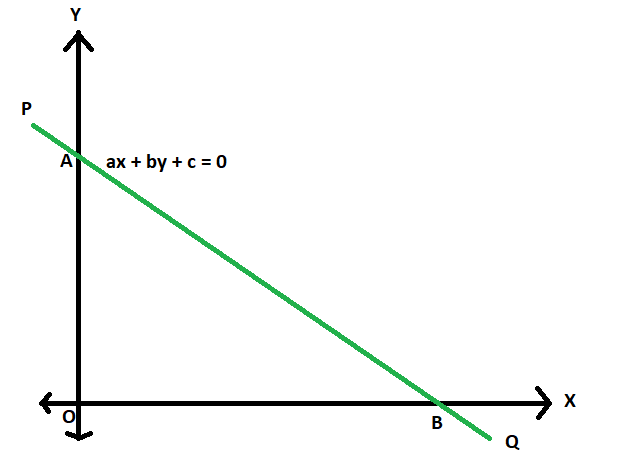# Area of triangle formed by the axes of co-ordinates and a given straight line

Given a straight line with equation coefficients as a, b & c(ax + by + c = 0), the task is to find the area of the triangle formed by the axes of co-ordinates and this straight line.

Examples:

```Input: a = -2, b = 4, c = 3
Output: 0.5625

Input: a = 4, b = 3, c = 12
Output: 6
```Approach:

1. Let PQ be the straight line having AB, the line segment between the axes.
The equation is,
ax + by + c = 0
2. so, in intercept form it can be expressed as,
x/(-c/a) + y/(-c/b) = 1
3. So, the x-intercept = -c/a
the y-intercept = -c/b
4. So, it is very clear now the base of the triangle AOB will be -c/a
and the base of the triangle AOB will be -c/b
5. So, area of the triangleBelow is the implementation of the above approach:

## C++

 `// C++ program area of triangle ` `// formed by the axes of co-ordinates ` `// and a given straight line ` ` `  `#include ` `using` `namespace` `std; ` ` `  `// Function to find area ` `double` `area(``double` `a, ``double` `b, ``double` `c) ` `{ ` `    ``double` `d = ``fabs``((c * c) / (2 * a * b)); ` `    ``return` `d; ` `} ` ` `  `// Driver code ` `int` `main() ` `{ ` `    ``double` `a = -2, b = 4, c = 3; ` `    ``cout << area(a, b, c); ` `    ``return` `0; ` `} `

## Java

 `// Java program area of triangle ` `// formed by the axes of co-ordinates ` `// and a given straight line ` ` `  `import` `java.io.*; ` ` `  `class` `GFG ` `{ ` ` `  `// Function to find area ` `static` `double` `area(``double` `a, ``double` `b, ``double` `c) ` `{ ` `    ``double` `d = Math.abs((c * c) / (``2` `* a * b)); ` `    ``return` `d; ` `} ` ` `  `// Driver code ` `public` `static` `void` `main (String[] args) ` `{ ` `     `  `    ``double` `a = -``2``, b = ``4``, c = ``3``; ` `    ``System.out.println(area(a, b, c)); ` `} ` `} ` ` `  `// This code is contributed by ajit. `

## Python3

 `# Python3 program area of triangle ` `# formed by the axes of co-ordinates ` `# and a given straight line ` ` `  `# Function to find area ` `def` `area(a, b, c): ` ` `  `    ``d ``=` `abs``((c ``*` `c) ``/` `(``2` `*` `a ``*` `b)) ` `    ``return` `d ` ` `  `# Driver code ` `a ``=` `-``2` `b ``=` `4` `c ``=` `3` `print``(area(a, b, c)) ` ` `  `# This code is contributed  ` `# by mohit kumar `

## C#

 `// C# program area of triangle ` `// formed by the axes of co-ordinates ` `// and a given straight line ` `using` `System; ` ` `  `class` `GFG ` `{ ` `     `  `// Function to find area ` `static` `double` `area(``double` `a, ``double` `b, ``double` `c) ` `{ ` `    ``double` `d = Math.Abs((c * c) / (2 * a * b)); ` `    ``return` `d; ` `} ` ` `  `// Driver code ` `static` `public` `void` `Main () ` `{ ` `     `  `    ``double` `a = -2, b = 4, c = 3; ` `    ``Console.WriteLine (area(a, b, c)); ` `} ` `} ` ` `  `// This code is contributed by akt_mit.  `

## PHP

 ` `

Output:

```0.5625
```

Attention reader! Don’t stop learning now. Get hold of all the important DSA concepts with the DSA Self Paced Course at a student-friendly price and become industry ready.

My Personal Notes arrow_drop_upCheck out this Author's contributed articles.

If you like GeeksforGeeks and would like to contribute, you can also write an article using contribute.geeksforgeeks.org or mail your article to contribute@geeksforgeeks.org. See your article appearing on the GeeksforGeeks main page and help other Geeks.

Please Improve this article if you find anything incorrect by clicking on the "Improve Article" button below.

Article Tags :
Practice Tags :

Be the First to upvote.

Please write to us at contribute@geeksforgeeks.org to report any issue with the above content.